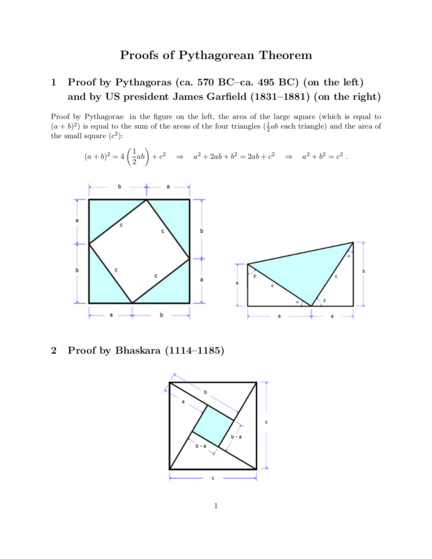# Proofs of Pythagoras TheoremContributed by:This pdf shows the proof of the Pythagoras theorem and from where it is derived to get through the basics. US President James Garfield has also illustrated the proof of the same.
1. Proofs of Pythagorean Theorem
1 Proof by Pythagoras (ca. 570 BC–ca. 495 BC) (on the left)
and by US president James Garfield (1831–1881) (on the right)
Proof by Pythagoras: in the figure on the left, the area of the large square (which is equal to
(a + b)2 ) is equal to the sum of the areas of the four triangles ( 21 ab each triangle) and the area of
the small square (c2 ):
 
2 1
(a + b) = 4 ab + c2 ⇒ a2 + 2ab + b2 = 2ab + c2 ⇒ a2 + b2 = c2 .
2
1
2. 3 Proof by similar triangles
Let CH be the perpendicular from C to the side AB in the right triangle 4ABC.
AB CB c a a2
Observation 1: 4ABC ∼ 4CBH, therefore = , i.e., = , hence e = . (1)
BC CH a e c
AB AC c b b2
Observation 2: 4ABC ∼ 4ACH, therefore = , i.e., = , hence d = . (2)
AC AH b d c
a2 b2
Finally, AB = BH + AH, i.e, c = e + d. Using (1) and (2), we rewrite this as c = + , which is
c c
equivalent to c2 = a2 + b2 .
The book
Elisha Scott Loomis, The Pythagorean Proposition: Its Demonstrations Analyzed and
Classified, and Bibliography of Sources for Data of the Four Kinds of “Proofs”, Second
edition, 1940, available at http://files.eric.ed.gov/fulltext/ED037335.pdf
contains 370 proofs of the Pythagorean Theorem.
2